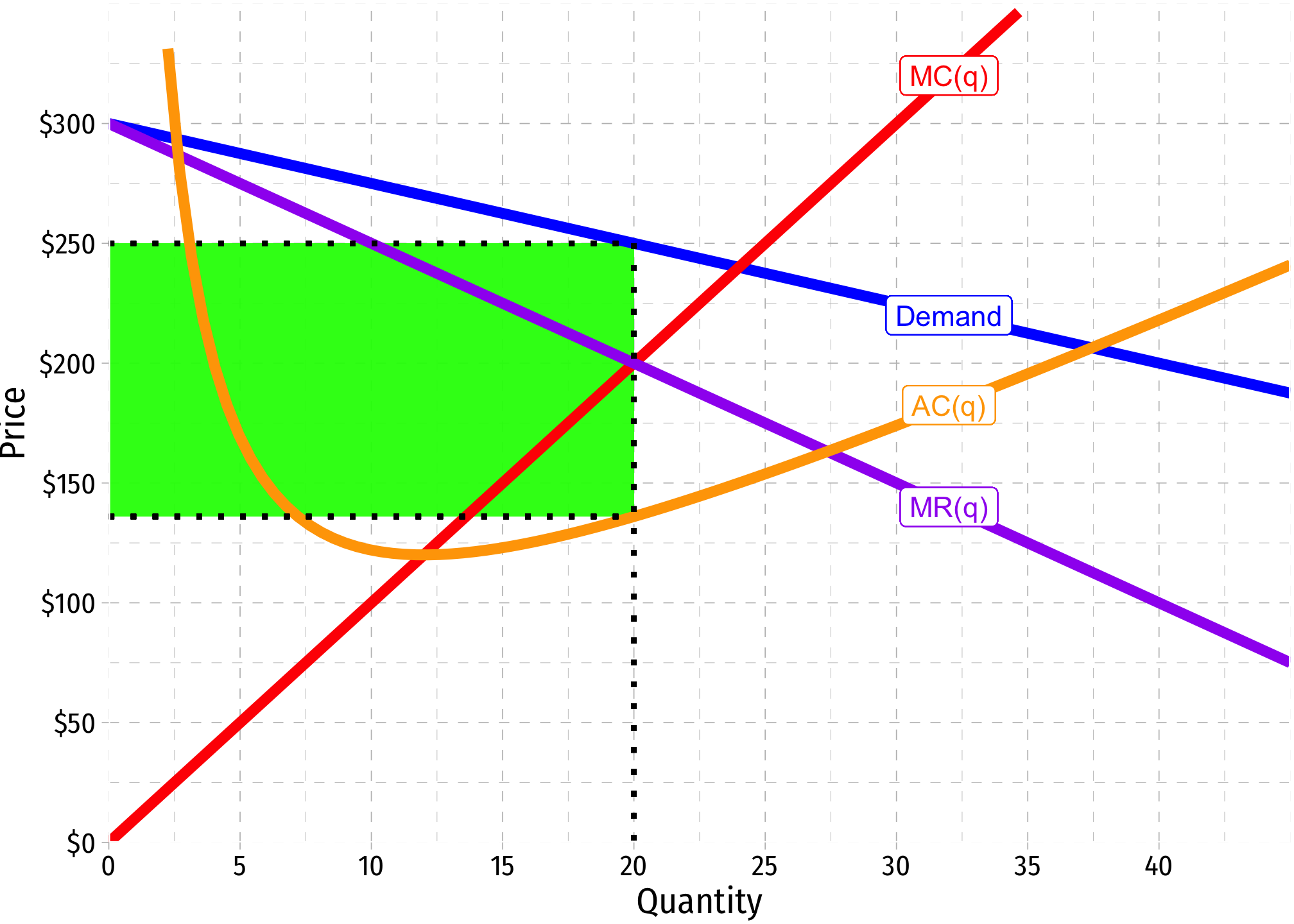Bob’s Bats produces baseball bats, and has the following costs: \begin{aligned} C(q)&=5q^2+720\\ MC(q)&=10q\\ \end{aligned}

and faces a market demand for bats: $q = 120-0.4p$

where quantity is measured in thousands of bats

### 1. Write Bob’s Marginal Revenue function.

If we can find the inverse demand (of the form $$p=a+bx$$, we can simply double the slope $$(b)$$ to get marginal revenue. We have the demand function, so solve it for $$p$$ to get the inverse:

\begin{align*} q &= 120-0.4p\\ q+0.4p&=120\\ 0.4p&=120-q\\ p&=300-2.5q\\ \end{align*}

This is the inverse demand function, so marginal revenue is

$MR(q)=300-5q$

### 2. Find the profit-maximizing quantity and price.

First, the quantity, we follow Rule #1 as always: the profit maximizing $$q^*$$ is where $$MR(q)=MC(q)$$

\begin{align*} MR(q)&= MC(q)\\ 300-5q&=10q\\ 300&=15q\\ 20&=q^\star\\ \end{align*}

Now that we know the profit-maximizing quantity, we need to find the maximum price consumers are willing to pay for 20 units. Plug this into the inverse demand function:

\begin{align*} p&=300-2.5q\\ p&=300-2.5(20)\\ p&=300-50\\ p^\star&=250\\ \end{align*}

### 3. How much total profit does Bob’s Bats earn? Should Bob stay or exit this industry in the long run?

Total profit again can be found with Rule #2: $$\pi=[p-AC(q)]q$$

We first need to find the Average Cost function from total cost, by dividing it by $$q$$:

$AC(q) = \frac{C(q)}{q} = \frac{5q^2+720}{q} = 5q+\frac{720}{q}$

Now we specifically need to find the average cost at 20 units:

\begin{align*} AC(q)&=5q+\frac{720}{q}\\ AC(20)&=5(20)+\frac{720}{(20)}\\ AC(20)&=100+36\\ AC(20)&=136\\ \end{align*}

Now just plug in the price, average cost, and quantity:

\begin{align*} \pi&=[p-AC(q)]q\\ \pi&=[250-136]20\\ \pi&=20\\ \pi&=2,280\\ \end{align*}

### 4. At what price would Bob’s Bats break even?

From before, we know that a firm’s break even price is at the minimum of its Average Cost curve, where Average Cost is equal to Marginal Cost. First let’s find the quantity where that happens:

\begin{align*} AC(q)&=MC(q)\\ 5q+\frac{720}{q}&=10q\\ \frac{720}{q}&=5q\\ 720&=5q^2\\ 144&=q^2\\ 12&=q\\ \end{align*}

This the quantity where AC is minimized and equal to MC. We need to find the price, so plug this quantity into either AC or MC. MC is easier here:

\begin{align*} MC(q)&=10q\\ MC(12)&=10(12)\\ MC(12)&=120\\ \end{align*}

### 6. Calculate the price elasticity of demand at Bob’s profit-maximizing price.

While you could calculate this manually, it’s a lot faster to use the full Lerner Index equation: $$L=\frac{p-MC(q)}{p}=-\frac{1}{\epsilon}$$. Since we know $$L$$, we can set it equal to $$-\frac{1}{\epsilon}$$ and solve for $$\epsilon$$:

\begin{align*} L&=-\frac{1}{\epsilon}\\ 0.20&=-\frac{1}{\epsilon}\\ 0.20\epsilon &=-1\\ \epsilon &=-\frac{1}{0.20}\\ \epsilon&=-5\\ \end{align*}

Demand is elastic. For every 1% the price increases (decreases), consumers will buy 5% less (more).